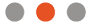# What is a Power Calculation?

The power of a statistical test is the probability that the test will reject the null hypothesis when the null hypothesis is false (i.e. the probability of not committing a Type II error, hence the probability of confirming the alternative hypothesis when the alternative hypothesis is true). The power is in general a function of the possible distributions, often determined by a parameter, under the alternative hypothesis. As the power increases, the chances of a Type II error occurring decrease. The probability of a Type II error occurring is referred to as the false negative rate (β). Therefore power is equal to 1 − β, which is also known as the sensitivity.

##TAKE THETUTORIALLet us walk you through the process of creating a trial